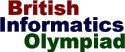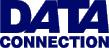The British Informatics Olympiad is the computing competition for schools and colleges. We are proud to present BIO'99.The 1999 British Informatics Olympiad - Round One
Worked solution to question 1

A digital river is a sequence of numbers where the number following n is n plus the sum of its digits. For example, 12345 is followed by 12360, since 1+2+3+4+5 = 15. If the first number of a digital river is k we will call it river k. ... Normal streams and rivers can meet, and the same is true for digital rivers. This happens when two digital rivers share some of the same values. For example: river 480 meets river 483 at 519, meets river 507 at 507, and never meets river 481.

1 (a). Every digital river will eventually meet river 1, river 3 or river 9. Write a program which inputs a single integer n (1<=n<=16384), and outputs the value where river n firstmeets one of these three rivers.

Sample run
River: 86
First meets river 1 at 101

Worked solution.

The first problem we must solve is to find the next number in the sequence. It is a good idea to write a function that does this as we will be calling it several times.

Given a starting point n, we begin with a variable s set to n, then add the sum of the digits of s to n. We do this by repeatedly dividing s by 10, adding the remainder to n each time, until s becomes zero. The remainder is calculated using mod in Pascal and the % operator in C or Java. Code to do this is given below.

 Pascal Java ```function next_river(n: integer): integer; var s: integer; begin s := n; while s > 0 do begin n := n + (s mod 10); s := s div 10; end; next_river := n; end;``` ```public int next_river( int n ) { int s = n; while( s > 0 ) { n = n + (s % 10); s = s / 10; } return n; }```

You can then call next_river(a) to find the next value in the sequence after a. It is straightforward to use this to follow a digital river.

The question is a little more complicated than that, however. We are told that if we follow rivers 1, 3 and 9 and any river far n enough, river n will meet one of the other three. (Proving this is not straightforward and is left to readers' curiosity.) This lets us note the following about the algorithm we will use to solve the problem.

• We need to store four things: the current value in river n, and the current values in river 1, river 3 and river 9. Appropriate starting values for these are n, 1, 3 and 9.
• Before every move we should check if river n has met one of the other three (for example, if we start with n=1 there is nothing to do).

The method I chose works as follows.

• Repeatedly move downstream in rivers 1, 3 and 9 until we reach or pass n.
• If we haven't reached a meeting point, move one step down river n.
• Keep doing this until we reach a meeting point.

Code that achieves this is as follows. It is assumed that n is declared elsewhere and is already set to the starting value.

Pascal

```procedure part1a;
var river1, river3, river9: integer;
begin
{ We will use these variables to follow the routes of rivers 1, 3 and 9 }
river1 := 1; river3 := 3; river9 := 9;

{ If we have not found a match, move along each river until we meet or
pass n.  If we still haven't met n, move one step along river n. }
while (n <> river1) and (n <> river3) and (n <> river9) do begin
while river1 < n do river1 := next_river( river1 );
while river3 < n do river3 := next_river( river3 );
while river9 < n do river9 := next_river( river9 );
if (n <> river1) and (n <> river3) and (n <> river9) then
n := next_river( n );
end;

{ Show the solution }
if river1 = n then writeln( 'First meets river 1 at ', n );
if river3 = n then writeln( 'First meets river 3 at ', n );
if river9 = n then writeln( 'First meets river 9 at ', n );
end;```

Java

```public void part1a() {
/* river1, river3 and river9 will be used to hold the current value
in river 1, river 3 and river 9 as we follow them along. */
int river1 = 1;
int river3 = 3;
int river9 = 9;

/* If we have not found a match, move along rivers 1, 3 and 9
until we meet or pass n.  If we still haven't got a meeting,
move one step along river n. */
while( (n != river1) && (n != river3) && (n != river9) ) {
while( river1 < n ) river1 = next_river( river1 );
while( river3 < n ) river3 = next_river( river3 );
while( river9 < n ) river9 = next_river( river9 );
if( (n != river1) && (n != river3) && (n != river9) )
n = next_river( n );
}
/* Show the solution */
if( river1 == n ) System.out.println( "First meets river 1 at " + n );
if( river3 == n ) System.out.println( "First meets river 3 at " + n );
if( river9 == n ) System.out.println( "First meets river 9 at " + n );
}```

All that is then required is to set up the program to read in n and call part1a.

A Java example program solving part 1(a) can be found in file bio99q1.java and can be viewed online using a Java-enabled browser - see the example solution program. The equivalent Turbo Pascal example program is in file bio99q1.pas, and a DOS executable of this program (compressed with PKZIP) is in bio99q1.zip.

Marking 1(a).

For each test of the program for 1(a) you need to type in a single integer. The response should be a statement containing two integers; there is  mark available for each correct integer. There are no marks for incorrect answers.

```  86     1  101
  108    9  117
  165    3  204
  852    3  1020
  999    9  999
  4759   1  10003
  13046  1  20014```

Additional marks are available for general program behaviour.

 Program inputs numbers.
 For each test a statement containing two numbers is output.
 Program terminates without crashing/hanging.

A total of  marks available for a correct solution to 1(a).1 (b). What is the lowest number that can be found in exactly 100 digital rivers? [For example, 8 is the lowest number in 4 rivers: rivers 1, 2, 4, and 8.]

The hardest part about this question is understanding what is meant by a number being 'in' several different rivers. In this example, 4 rivers include the value 8: river 8, river 4 (with the values 4, 8, ...), river 2 (going 2, 4, 8, ...) and river 1 (1, 2, 4, 8, ...). Each of these is considered to be a different river. In this case, they all happen to follow from river 1, but that will not be the case in general because rivers merge.

The way to solve this is to count the times each number is met as we follow every river in turn. We start with an array hits[], initially set to zeros. We follow river 1, incrementing the appropriate element of hits[] for each point in river 1, stopping at some end value. We then follow river 2, then river 3, and so on, in the same way, stopping when we've followed all the rivers up to our chosen terminating value. We can then check to find which number is the lowest that it an element in exactly 100 different rivers.

I chose 500 as the end point, which just happened to be large enough. If you'd started with a value smaller than the correct answer, 416, the program would not have found a solution and you would have known to try a bigger end value.

This can be achieved using the following code, which calls next_river().

Pascal

```procedure part1b;
var start: integer;
current: integer;
hits: array[1..500] of integer;
begin
{ hits will store the number of times we have reached each river. }
for current := 1 to 500 do hits[current] := 0;

{ Follow rivers 1 to 500 and note each time a value is 'hit' }
for start := 1 to 500 do begin
current := start;
while current < 500 do begin
inc(hits[current]);
current := next_river( current );
end;
end;

{ Find the first time we meet a value 100 times }
for current := 1 to 500 do if hits[current] = 100 then begin
writeln( 'First number in 100 rivers is ', current );
break;
end;
end;```

Java

```public void part1b() {
int start;  /* Current starting river */
int current;   /* Position in river start */
int[] hits = new int;  /* Number of times we've met each value */

/* Initialise number of hits to zero */
for( current = 1; current < 500; current++ ) hits[current] = 0;

/* Follow rivers 1 to 500 */
for( start = 1; start < 500; start++ ) {
current = start;
while( current < 500 ) {
hits[current]++;
current = next_river( current );
}
}

/* Find the first time we meet a number 100 times */
for( current = 1; current < 500; current++ )
if( hits[current] == 100 ) {
System.out.println( "First number in 100 rivers is " + current );
break;
}
}```

Java code solving parts 1(b) is also given in file bio99q1.java. The equivalent Turbo Pascal example program is in file bio99q1.pas, and a DOS executable of this program (compressed with PKZIP) is in bio99q1.zip.

Marking 1(b).

The correct answer, which gets  marks, is 416. Additionally, a number of answers, obtained through making incorrect assumptions, can get partial marks: the answer 427 is worth  marks, and the answer 418 is worth .

The British Informatics Olympiad